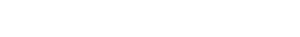# Beyond Perception: Exploring the Secrets of Organism Size | Cambridge IGCSE Biology[Please watch the video attached at the end of this blog for a visual explanation on Beyond Perception: Exploring the Secrets of Organism Size]

Topic of organisation of an organism and subtopic of “Size of Specimens”. So  in this video we are going to  be covering the following learning outcomes.

1. State and use the formula: magnification = image size ÷ actual size
2. Calculate magnification and size of biological specimens using millimetres as units
3. Convert measurements between millimetres (mm) and micrometres (μm)

In this article, we will focus on the magnification formula and how to answer questions in regard to magnification.

Before diving into the calculations, let us first try to understand what magnification is all about. In Biology, many of the structures we study about are very small. For example, we learnt all about cells and cell structures and the different types of cells in plants and animals and so on. By now we have understood that cells are extremely tiny structures that we cannot see by our eyes alone. If anyone wants to observe cells, we would have to use a microscope.

There are two types of microscopes which are the light microscope and the more advanced electron microscope that helps us to see the cells and their parts.

### What do light microscopes and electron microscopes do?

When we place the tiny biological structure under the microscope, the microscope enlarges that structure and shows it to us as an enlarged image. So what we see through the microscope is an enlarged image of the tiny little structure.

Light microscopes can magnify objects up to 1000 times, while electron microscopes can magnify more than 200,000 times of the actual size.

So that’s basically what magnification is all about: magnification is simply how much an actual object has been enlarged by. For example the light microscope can enlarge an object by roughly 1000 times of the actual size. So that is what magnification is and we give magnification by this formula:When we try out different questions we can put this into a formula triangle, where we have image size at the top (I) and actual size (A) and magnification (M) at the bottom. A stands for actual size and M for magnification.Tips and Pointers when attempting Questions dealing with size and magnification

1. Always look at the units that have been given in the question.
Sometimes there will be questions where they give the image size and actual size in different units. Therefore if the units are not converted to one, the answer we get will be a wrong answer. So only continue once it is verified both are in the same unit.

2. Learn the equation triangle for magnification,
Learn the formula triangle of magnification, where there is image size at the top, and actual size and magnification at the bottom. Learn this triangle and if a question is given regarding this, write this on the page straight away, immediately draw up the formula triangle

3. Magnification does not have a unit,
Magnification does not have a unit, it is just written as times the number (for example, times 500 (✕500) or times 10 (✕10), etc.

4. The relationship between cm and mm, mm and μm
Also when we are dealing with these questions, we sometimes need to convert between units. If we are dealing with centimetres and millimetres, remember the relationship between them. And if there is a question with micrometres, remember the relationship between them too.Practice Question 1:

The actual thickness of the leaf diagram is 2000μm , but the image size of the leaf in the diagram is 50 mm. What is the magnification of the diagram?Actual thickness:  2000μm

Image size: 50 mm

One important thing that needs to be noticed is that the actual size is given in micrometres whereas the image size is given in millimetres. We need to convert one into the other’s units.

We can convert 2000μm into millimetres.

2000  ÷ 1000 = 2mm.

Therefore the actual size of the image is 2mm.

We can then insert them into the magnification formula:

Magnification   = Image Size ÷ Actual Size

= 50mm ÷ 2mm

= 25 (no units)

There is another example in the video for you to attempt and check out!

Revising Beyond Perception: Exploring the Secrets of Organism Size

Study the formula triangle and pay attention to the units given in the question. Ensure that all the units of the measurements are the same before solving the question.

Some questions on organism size can be found here as well, and you can time your answers to see if you can stick to the time limit given.

If you are struggling with IGCSE revision or Biology in particular, you can reach out to us at Tutopiya to join revision sessions or find yourself the right tutor for you.

Attempt the quiz to know where you stand!